## Students of Grade 9, planned to plant saplings along straight lines, parallel to each other to one side of the playground ensuring that they had enough play area. Let us assume that they planted one of the rows of the saplings along the line 𝑦 = 𝑥 − 4. Let L be the set of all lines which are parallel on the ground and R be a relation on L.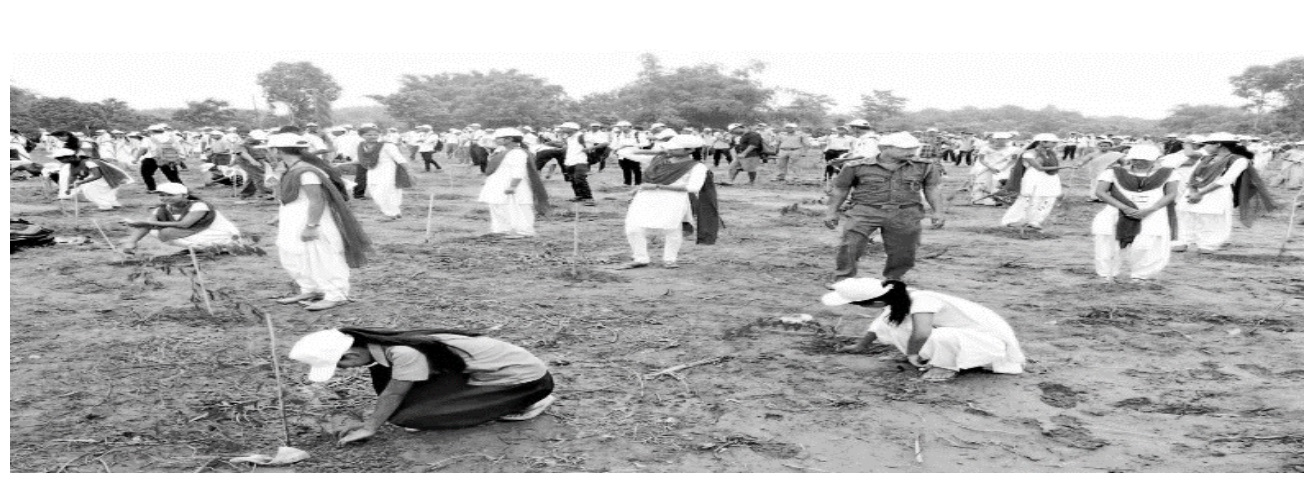(adsbygoogle = window.adsbygoogle || []).push({}); Answer the following using the above information.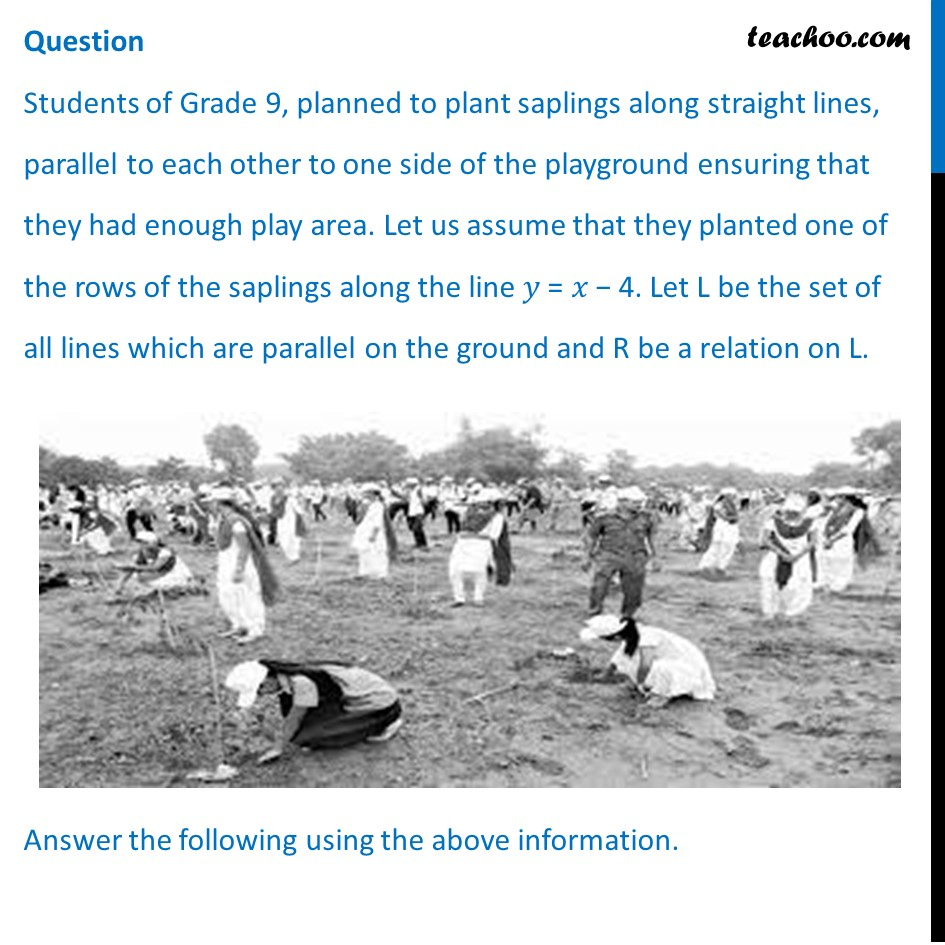## Let relation R be defined by R = {(L 1 , L 2 ) : L 1 ∥ L 2 where L 1 , L 2 ∈ L} then R is______ relation (a) Equivalence (b) Only reflexive (c) Not reflexive (d) Symmetric but not transitive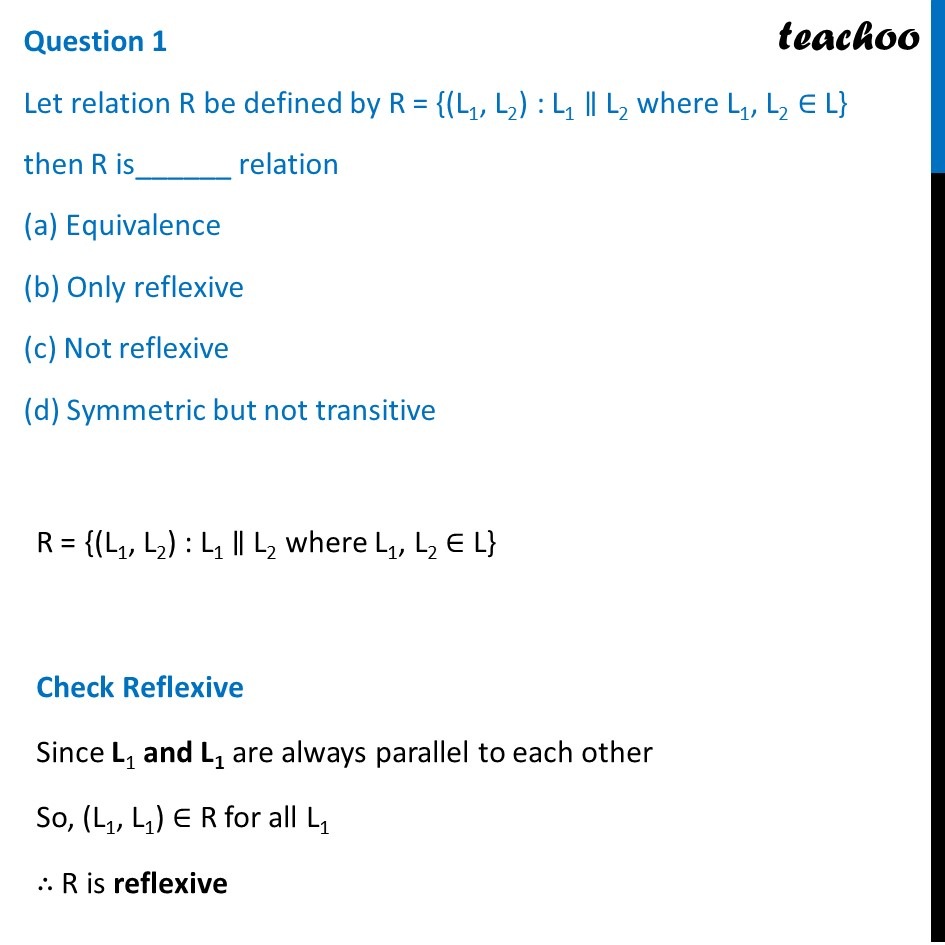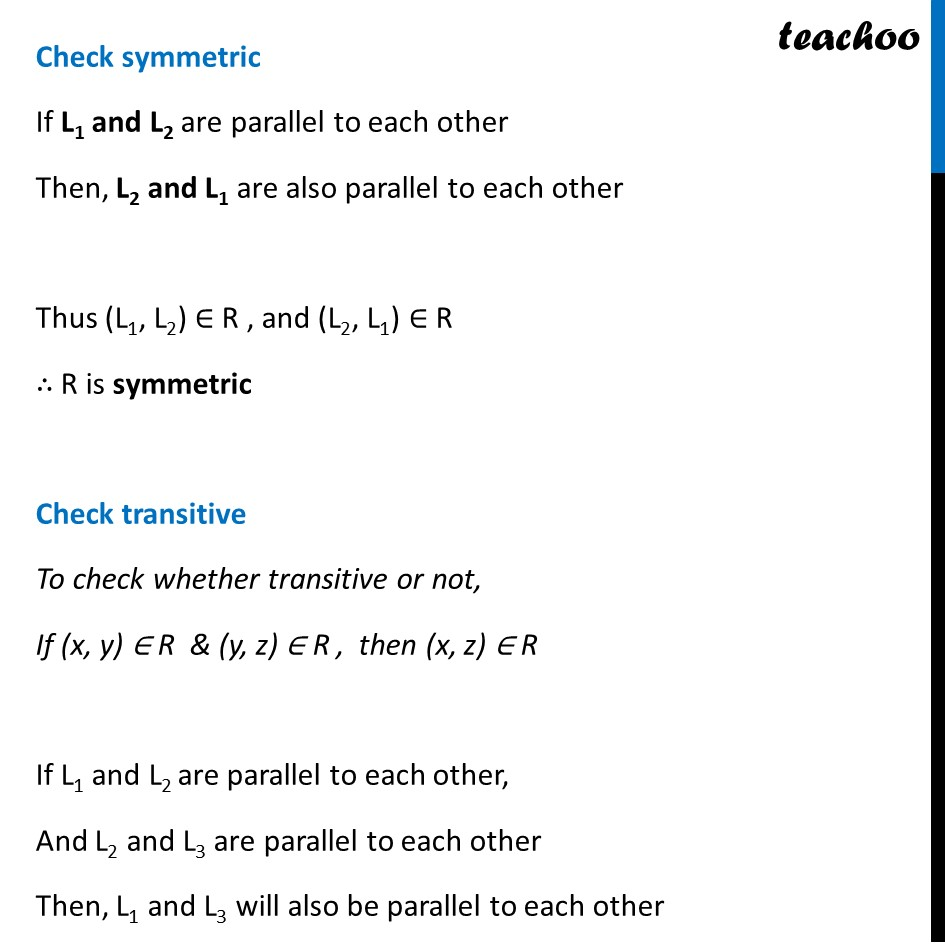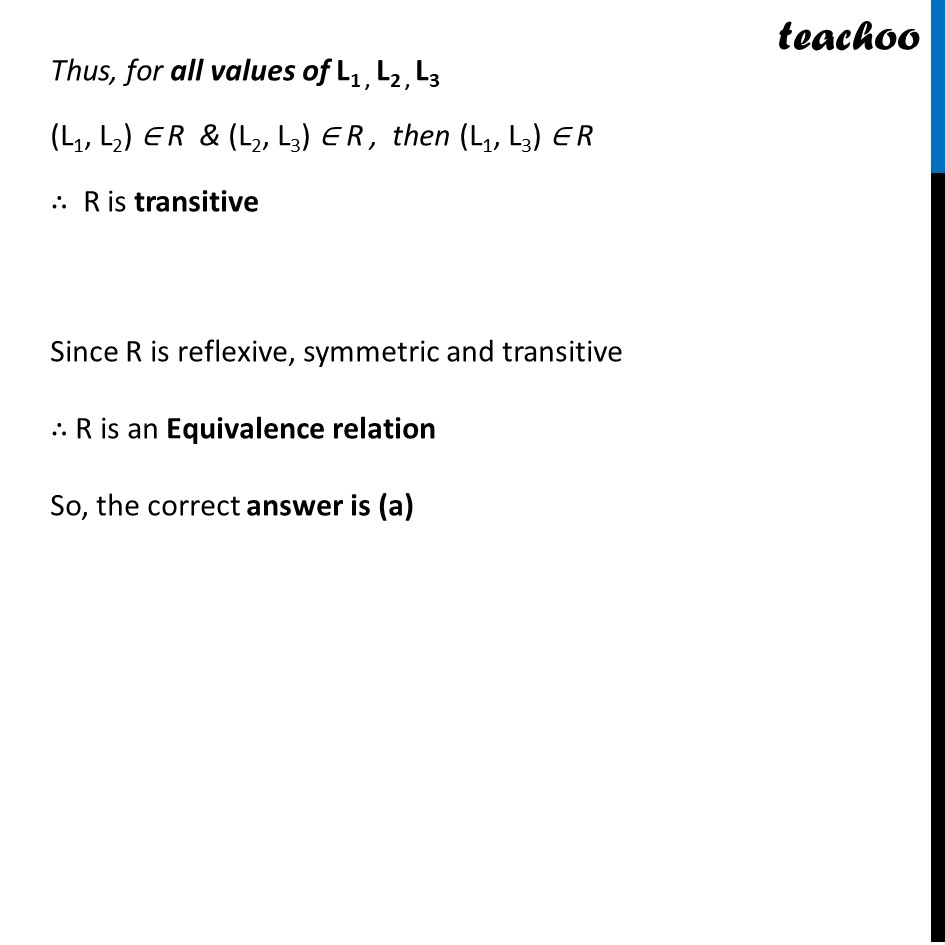## Let R = {(L 1 , L 2 ) ∶ L 1 ⊥ L 2 where L 1 , L 2 ∈ L} which of the following is true? (a) R is Symmetric but neither reflexive nor transitive (b) R is Reflexive and transitive but not symmetric (c) R is Reflexive but neither symmetric nor transitive (d) R is an Equivalence relation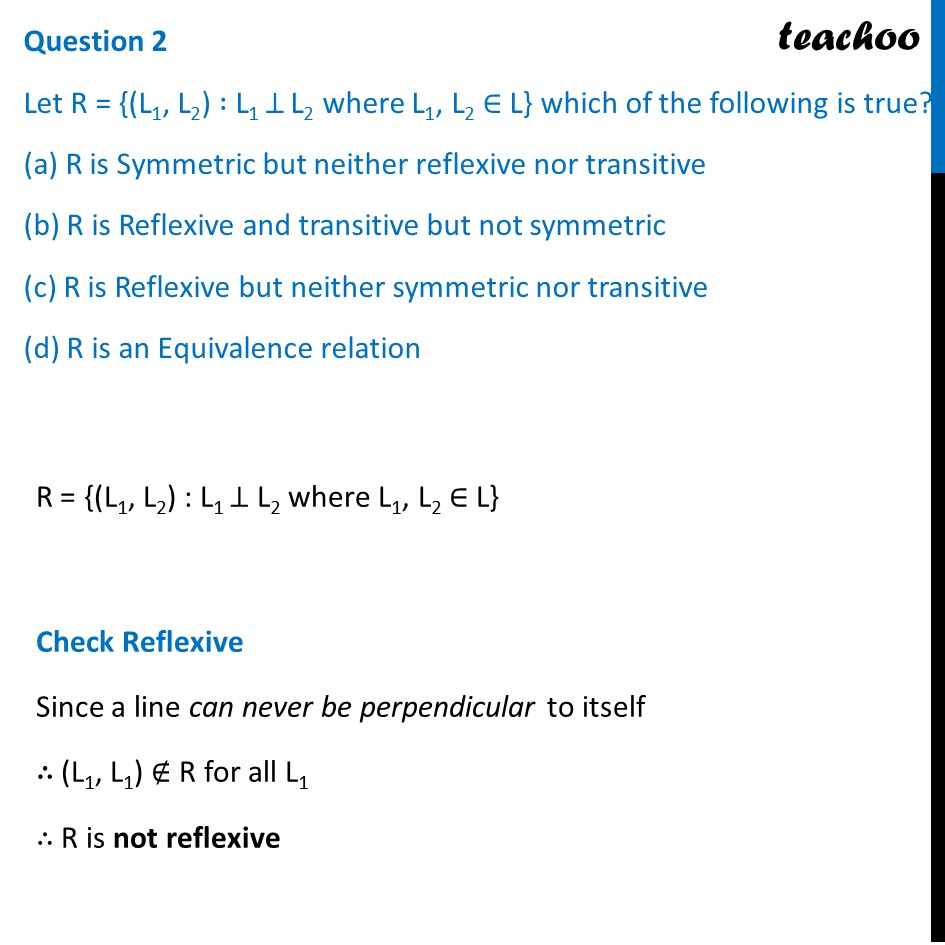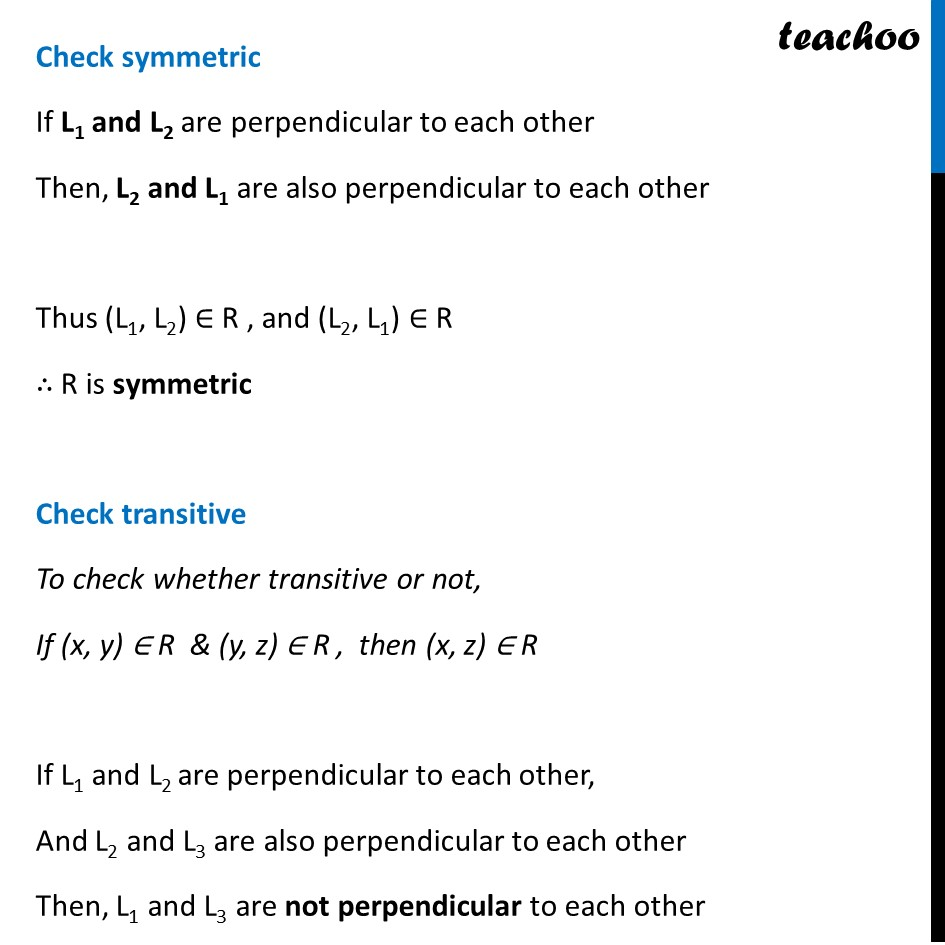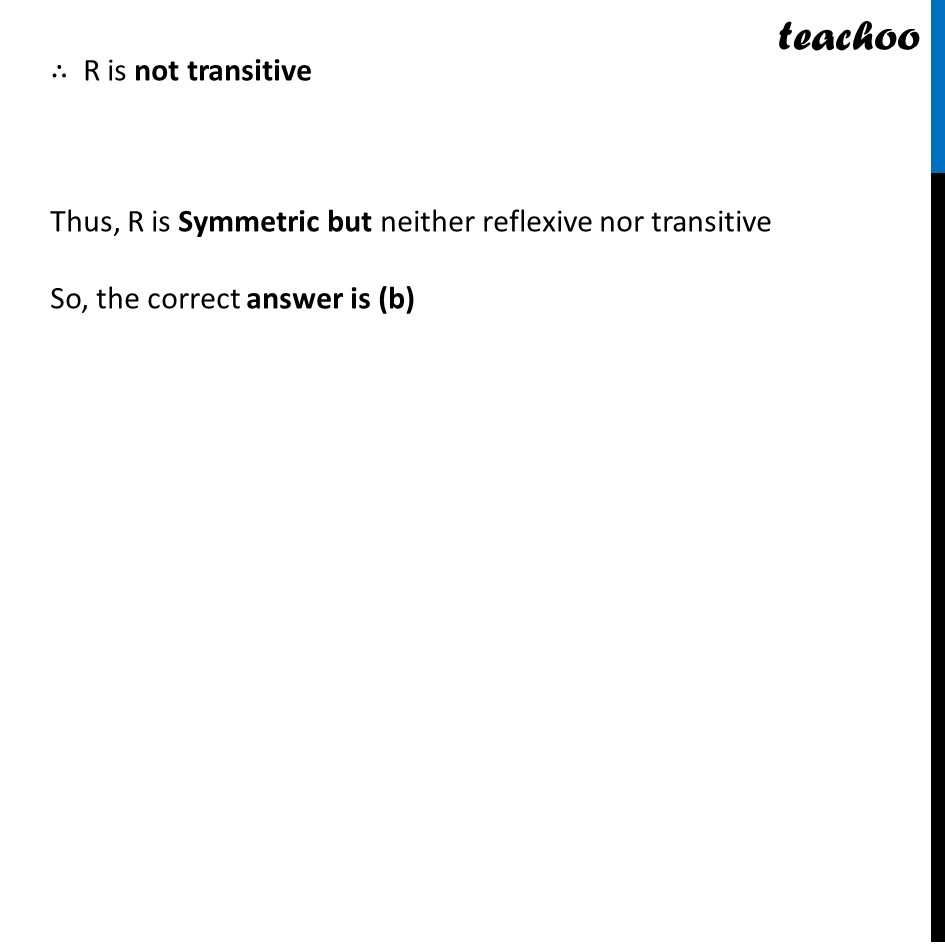## The function f: R → R defined by 𝑓(𝑥) = 𝑥 − 4 is___________ (a) Bijective (b) Surjective but not injective (c) Injective but not Surjective (d) Neither Surjective nor Injective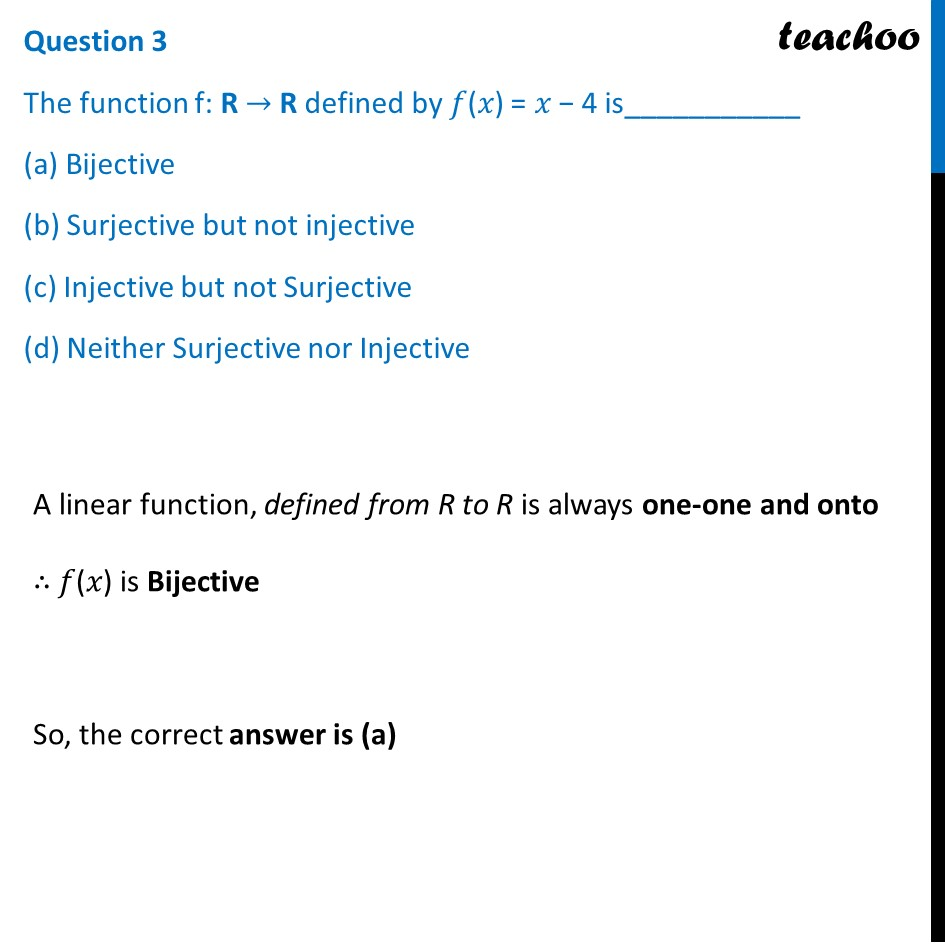For Proof, Check Example 2 – Chapter 1 Class 12

## Let 𝑓: 𝑅 → 𝑅 be defined by 𝑓(𝑥) = 𝑥 − 4. Then the range of 𝑓(𝑥) is ________ (a) R (b) Z (c) W (d) Q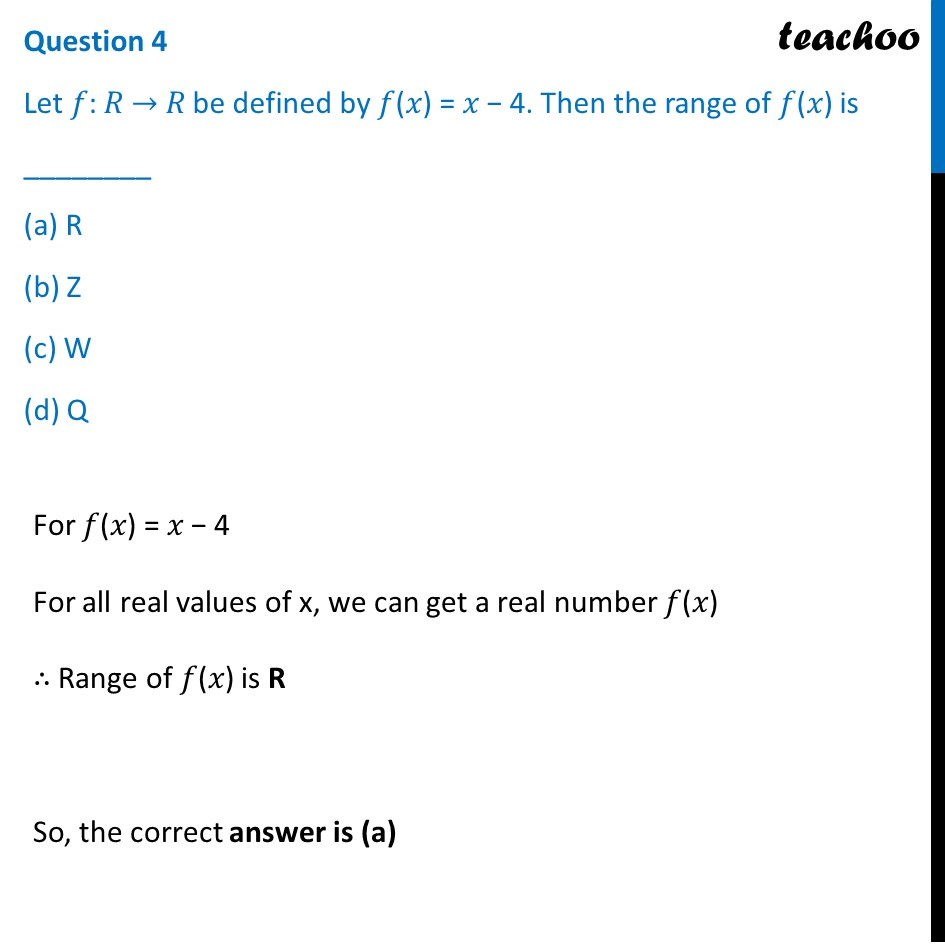## Let R = {(L 1 , L 2 ) : L 1 is parallel to L 2 and L 1 : y = x – 4} then which of the following can be taken as L 2 ? (a) 2x – 2y + 5 = 0 (b) 2x + y = 5 (c) 2x + 2y + 7 = 0 (d) x + y = 7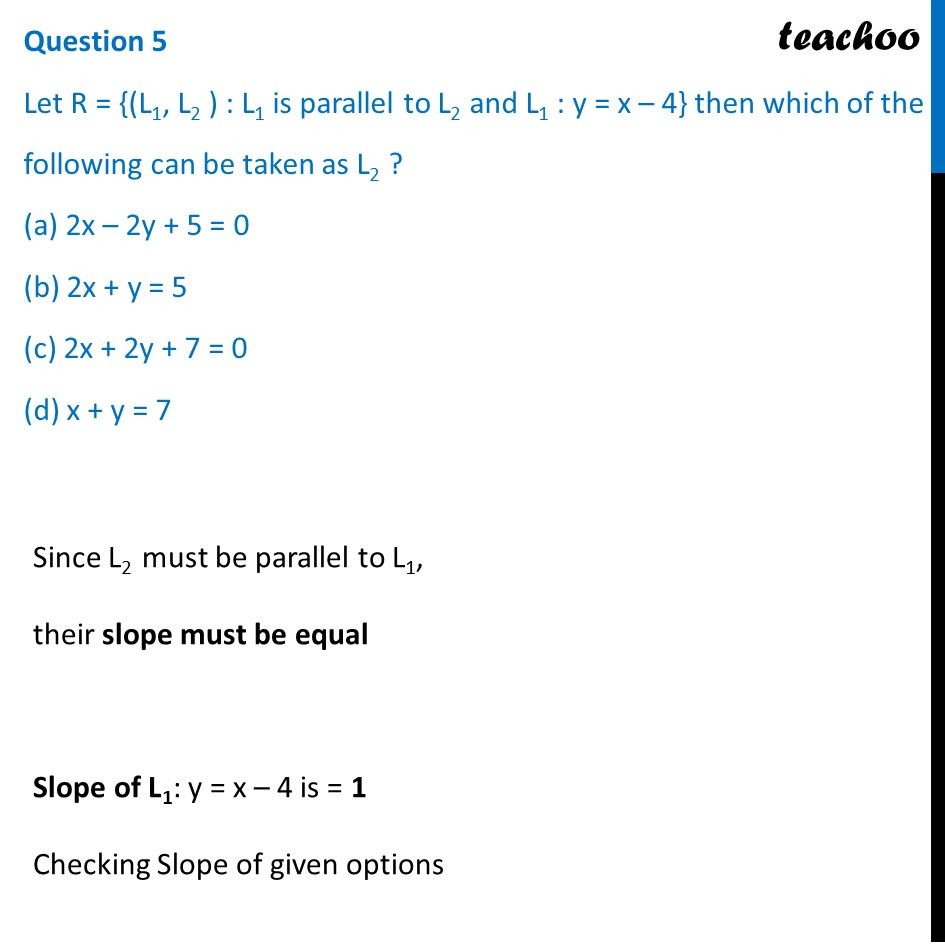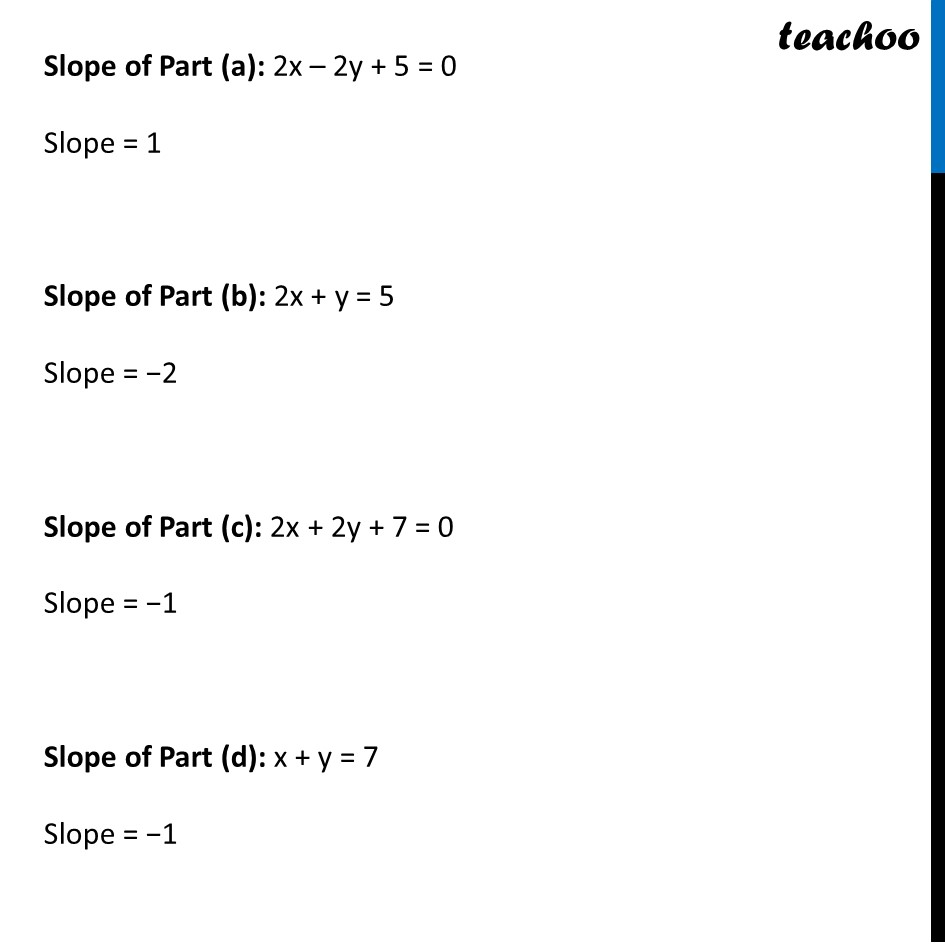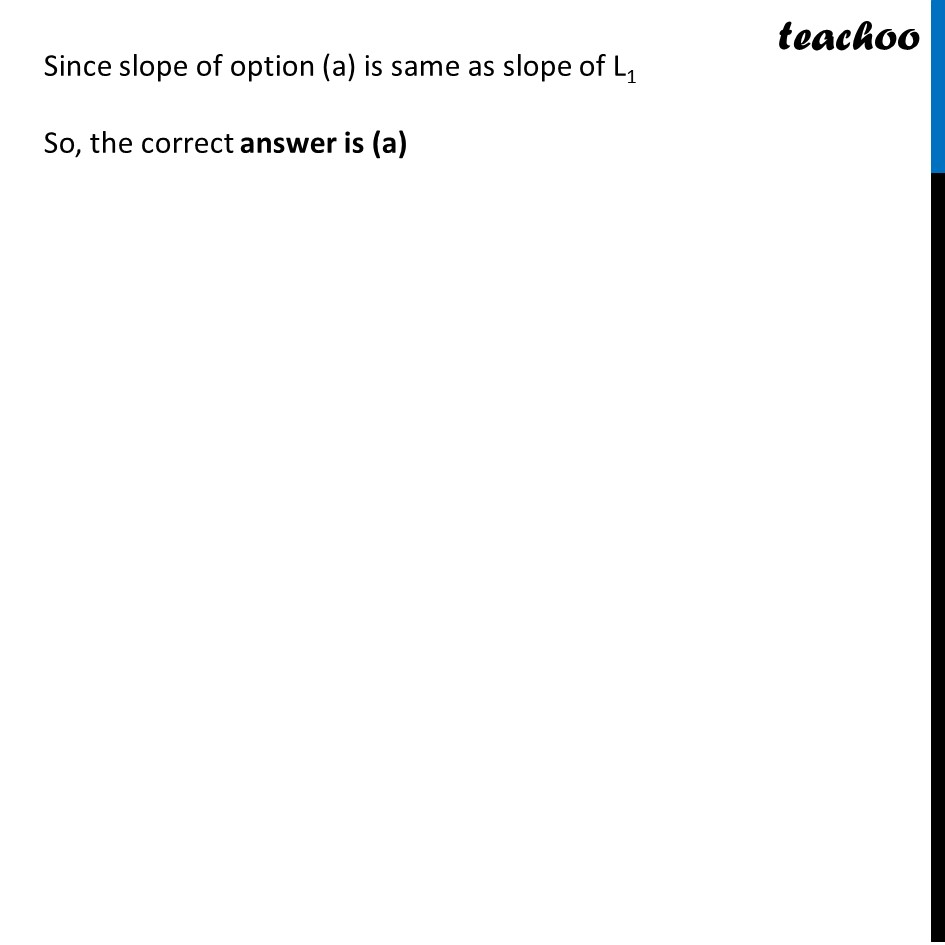1. Chapter 1 Class 12 Relation and Functions (Term 1)
2. Serial order wise
3. Case Based Questions (MCQ)

Transcript

Question Students of Grade 9, planned to plant saplings along straight lines, parallel to each other to one side of the playground ensuring that they had enough play area. Let us assume that they planted one of the rows of the saplings along the line 𝑦 = 𝑥 − 4. Let L be the set of all lines which are parallel on the ground and R be a relation on L. Answer the following using the above information. Question 1 Let relation R be defined by R = {(L1, L2) : L1 ∥ L2 where L1, L2 ∈ L} then R is______ relation (a) Equivalence (b) Only reflexive (c) Not reflexive (d) Symmetric but not transitive R = {(L1, L2) : L1 ∥ L2 where L1, L2 ∈ L} Check Reflexive Since L1 and L1 are always parallel to each other So, (L1, L1) ∈ R for all L1 ∴ R is reflexive Check symmetric If L1 and L2 are parallel to each other Then, L2 and L1 are also parallel to each other Thus (L1, L2) ∈ R , and (L2, L1) ∈ R ∴ R is symmetric Check transitive To check whether transitive or not, If (x, y) ∈ R & (y, z) ∈ R , then (x, z) ∈ R If L1 and L2 are parallel to each other, And L2 and L3 are parallel to each other Then, L1 and L3 will also be parallel to each other Thus, for all values of L1 , L2 , L3 (L1, L2) ∈ R & (L2, L3) ∈ R , then (L1, L3) ∈ R ∴ R is transitive Since R is reflexive, symmetric and transitive ∴ R is an Equivalence relation So, the correct answer is (a) Question 2 Let R = {(L1, L2) ∶ L1 ⊥ L2 where L1, L2 ∈ L} which of the following is true? (a) R is Symmetric but neither reflexive nor transitive (b) R is Reflexive and transitive but not symmetric (c) R is Reflexive but neither symmetric nor transitive (d) R is an Equivalence relation R = {(L1, L2) : L1 ⊥ L2 where L1, L2 ∈ L} Check Reflexive Since a line can never be perpendicular to itself ∴ (L1, L1) ∉ R for all L1 ∴ R is not reflexive Check symmetric If L1 and L2 are perpendicular to each other Then, L2 and L1 are also perpendicular to each other Thus (L1, L2) ∈ R , and (L2, L1) ∈ R ∴ R is symmetric Check transitive To check whether transitive or not, If (x, y) ∈ R & (y, z) ∈ R , then (x, z) ∈ R If L1 and L2 are perpendicular to each other, And L2 and L3 are also perpendicular to each other Then, L1 and L3 are not perpendicular to each other ∴ R is not transitive Thus, R is Symmetric but neither reflexive nor transitive So, the correct answer is (b) Question 3 The function f: R → R defined by 𝑓(𝑥) = 𝑥 − 4 is___________ (a) Bijective (b) Surjective but not injective (c) Injective but not Surjective (d) Neither Surjective nor Injective A linear function, defined from R to R is always one-one and onto ∴ 𝑓(𝑥) is Bijective So, the correct answer is (a) Question 4 Let 𝑓: 𝑅 → 𝑅 be defined by 𝑓(𝑥) = 𝑥 − 4. Then the range of 𝑓(𝑥) is ________ (a) R (b) Z (c) W (d) Q For 𝑓(𝑥) = 𝑥 − 4 For all real values of x, we can get a real number 𝑓(𝑥) ∴ Range of 𝑓(𝑥) is R So, the correct answer is (a) Question 5 Let R = {(L1, L2 ) : L1 is parallel to L2 and L1 : y = x – 4} then which of the following can be taken as L2 ? (a) 2x – 2y + 5 = 0 (b) 2x + y = 5 (c) 2x + 2y + 7 = 0 (d) x + y = 7 Since L2 must be parallel to L1, their slope must be equal Slope of L1: y = x – 4 is = 1 Checking Slope of given options Slope of Part (a): 2x – 2y + 5 = 0 Slope = 1 Slope of Part (b): 2x + y = 5 Slope = −2 Slope of Part (c): 2x + 2y + 7 = 0 Slope = −1 Slope of Part (d): x + y = 7 Slope = −1 Since slope of option (a) is same as slope of L1 So, the correct answer is (a)

Case Based Questions (MCQ)